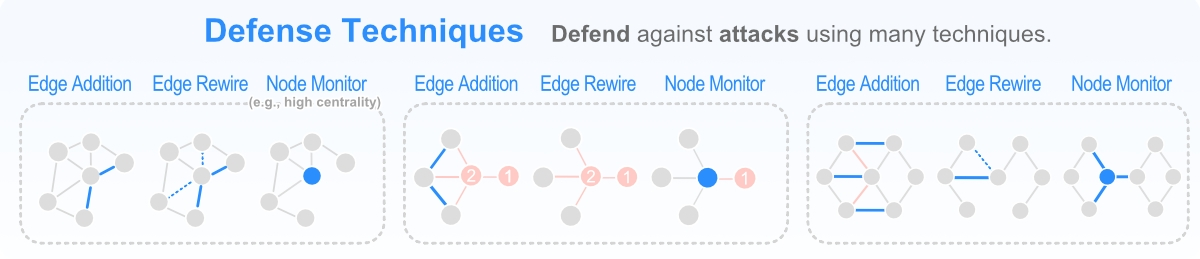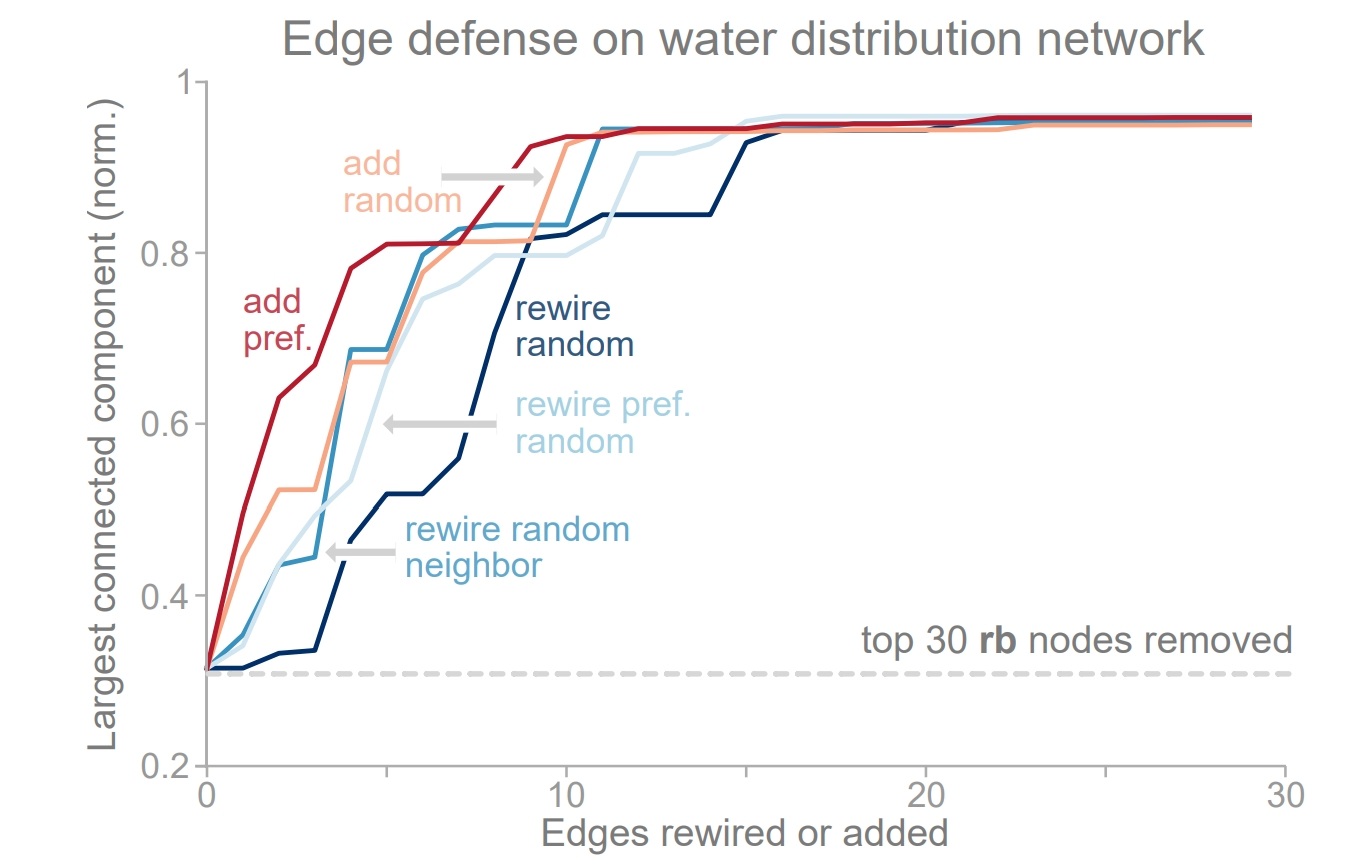# Tutorial 3: Defending a Network¶

The same centrality measures that are effective in attacking a network, are important to network defense (e.g., degree, betweenness, PageRank,eigenvector, etc.). In fact, if an attack strategy is known a priori, node monitoring can largely prevent an attack altogether. Below, we provide a high-level overview of several heuristic and optimization based defense techniques. Then, we show several defense techniques can be used to robustify an attacked network.There are 3 common ways to defend a network: edge addition, edge rewiring and node monitoring. Selecting an ideal defense requires knowledge of the network topology and some information on the expected attack (or failure).

We categorize defense techniques based on whether they operate heuristically, modifying graph structure independent of a robustness measure, or by optimizing for a particular robustness measure. Within each category a network can be defended i.e., improve its robustness by: (1) edge rewiring, (2) edge addition, or (iii) node monitoring. Edge rewiring is considered a low cost, less effective version of edge addition. On the other hand, edge addition almost always provides stronger defense. Node monitoring provides an orthogonal mechanism to increase network robustness by monitoring (or removing) nodes in the graph. This has an array of applications, including: (i) preventing targeted attacks, (ii) mitigating cascading failures, and (iii) reducing the spread of network entities. Below, we highlight several heuristic edge rewiring and addition techniques contained in TIGER:

• Random addition: adds an edge between two random nodes.

• Preferential addition: adds an edge connecting two nodes with the lowest degrees.

• Random edge rewiring: removes a random edge and adds one using (1).

• Random neighbor rewiring: randomly selects neighbor of a node and removes the edge. An edge is then added using (1).

• Preferential random edge rewiring: selects an edge, disconnects the higher degree node, and reconnects to a random one.

To help users evaluate the effectiveness of defense techniques, we compare 5 edge defenses on the Kentucky KY-2 water distribution network, averaged over 10 runs, using the code below.

```import os
import sys
import matplotlib.pyplot as plt
from collections import defaultdict

from graph_tiger.graphs import graph_loader
from graph_tiger.defenses import Defense

def plot_results(graph, steps, results, title):
plt.figure(figsize=(6.4, 4.8))

for method, result in results.items():
result = [r / len(graph) for r in result]
plt.plot(list(range(steps)), result, label=method)

plt.ylim(0, 1)
plt.ylabel('LCC')
plt.xlabel('N_rm / N')
plt.title(title)
plt.legend()

save_dir = os.getcwd() + '/plots/'
os.makedirs(save_dir, exist_ok=True)

plt.savefig(save_dir + title + '.pdf')
plt.show()
plt.clf()

graph = graph_loader(graph_type='water', seed=1)

params = {
'runs': 10,
'steps': 30,
'seed': 1,

'k_a': 30,
'attack': 'rb_node',
'attack_approx': int(0.1*len(graph)),

'defense': 'rewire_edge_preferential',

'plot_transition': False,
'gif_animation': False,

'edge_style': None,
'node_style': None,
'fa_iter': 20
}

edge_defenses = ['rewire_edge_random', 'rewire_edge_random_neighbor', 'rewire_edge_preferential_random', 'add_edge_random', 'add_edge_preferential']

print("Running edge defenses")
results = defaultdict(str)
for defense in edge_defenses:
params['defense'] = defense

a = Defense(graph, **params)
results[defense] = a.run_simulation()
plot_results(graph, params['steps'], results, title='water:edge_defense_runs={},attack={},'.format(params['runs'], params['attack']))
```

The results of the code are shown in the figure below, where the network is initially attacked using the RB attack strategy (30 nodes removed), and the success of each defense is measured based on how it can reconnect the network by adding or rewiring edges in the network (higher is better). Based on the figure, we identify three key observations: (i) preferential edge addition performs the best; (ii) edge addition, in general, outperforms rewiring strategies; and (iii) random neighbor rewiring typically performs better than the other rewiring strategies.Comparing ability of 5 edge defenses to improve KY-2 network robustness after removing 30 nodes via RB attack. Edge addition performs the best, with random edge rewiring performing the worst.